# 详解CNN的卷积层, 采样层, 全连接层的作用 | 浅书 - AI学习笔记

## 卷积神经网络概览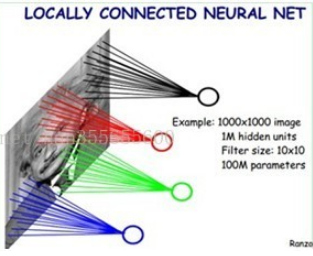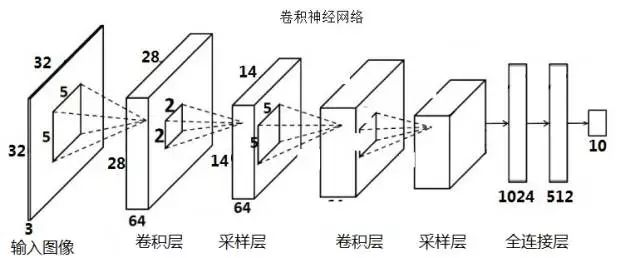## 卷积层

### 卷积运算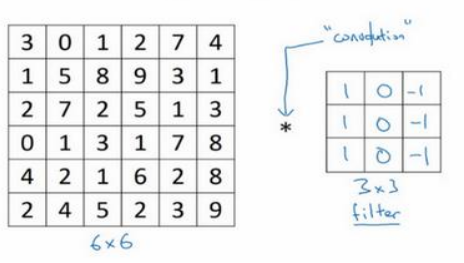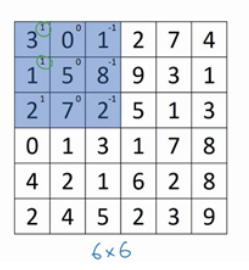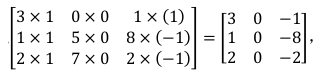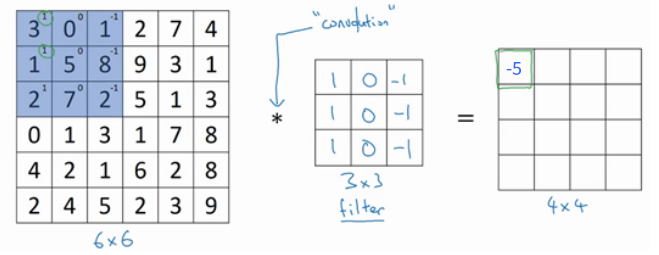0 × 1 + 5 × 1 + 7 × 1 + 1 × 0 + 8 × 0 + 2 × 0 + 2 × (-1) + 9 × (-1) + 5 × (-1) = -4 写在刚刚得到的-5的右边。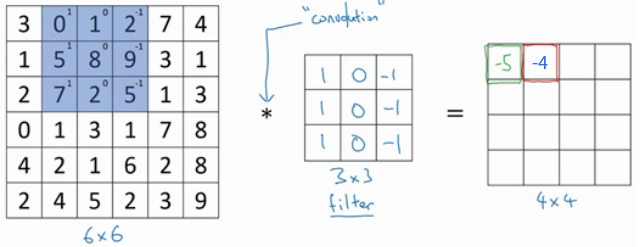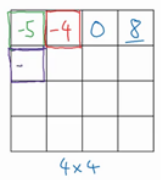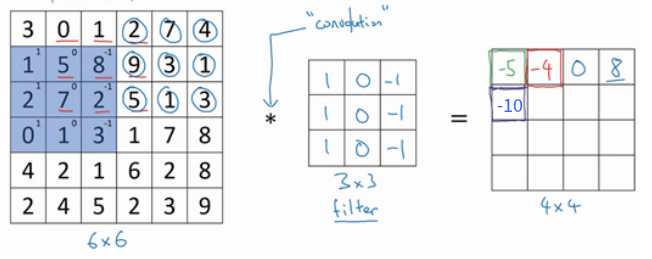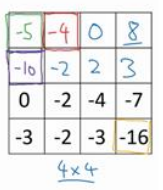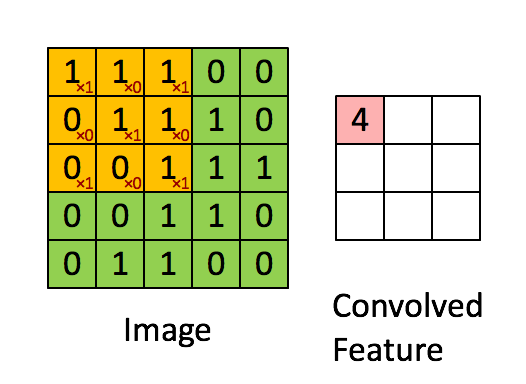### 过滤器(filter)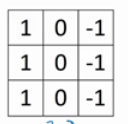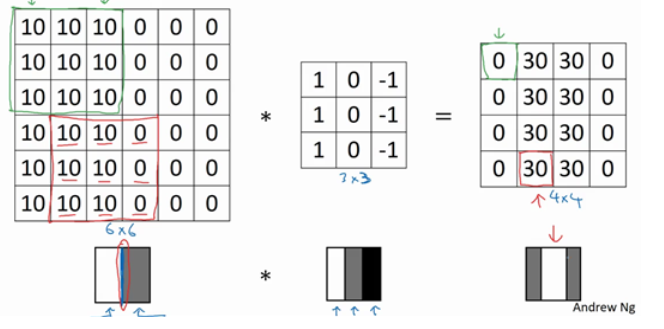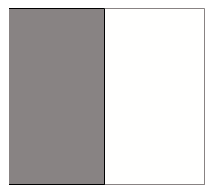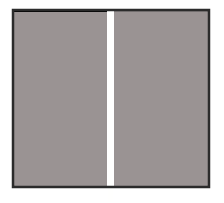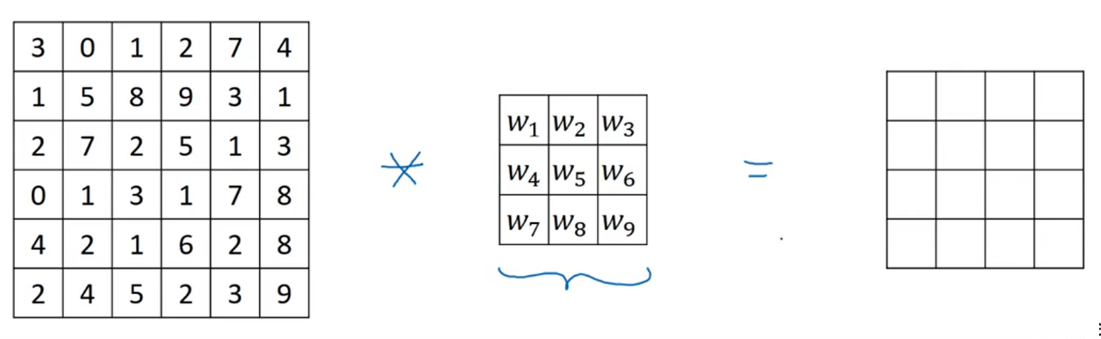### 卷积滑动步长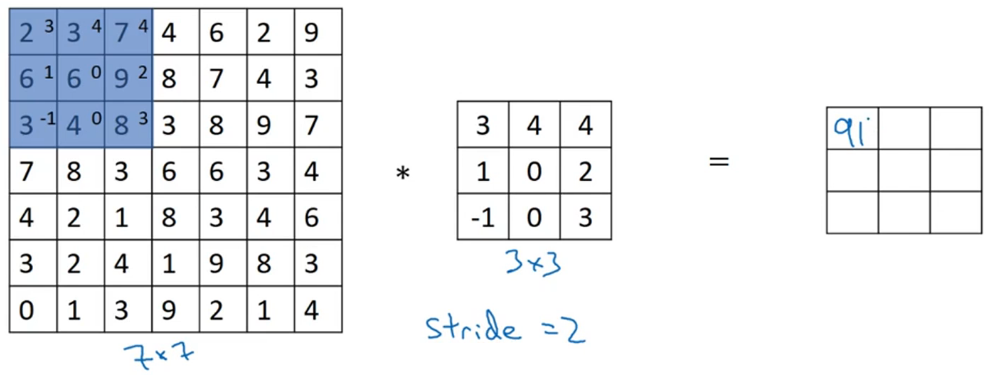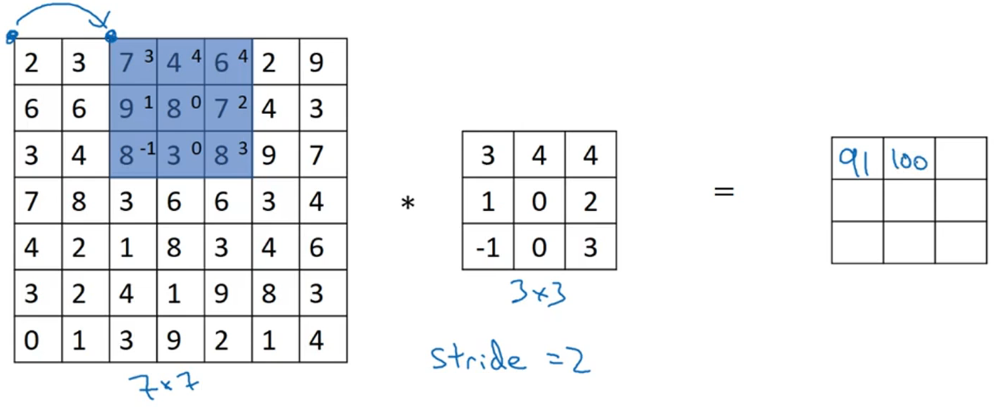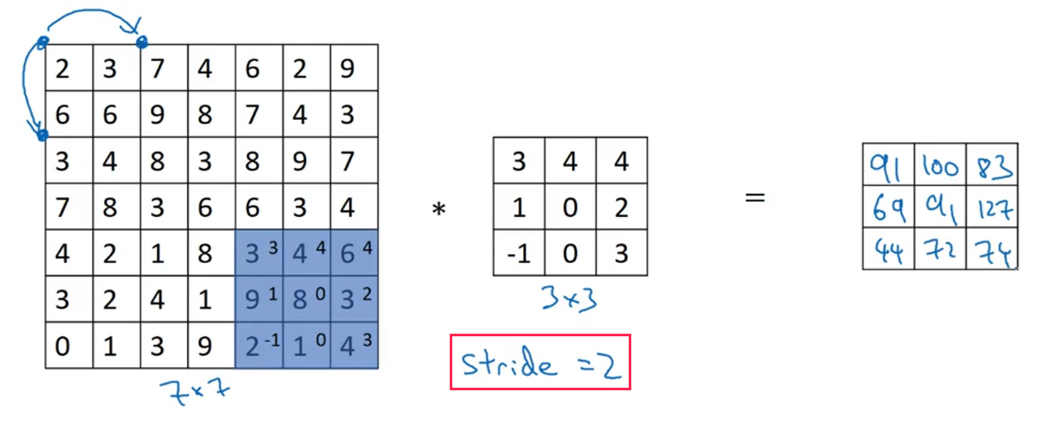( n - f ) / s + 1

( 7 - 3 ) / 2 + 1 = 3

( 6 - 3 ) / 1 + 1 = 4

1. 每次卷积之后图像就会缩小。
2. 图片的中间部分进行了多次卷积，而边缘部分的数据则参与卷积的次数较少，意味着你丢掉了图像边缘位置的许多信息。

### Zero Padding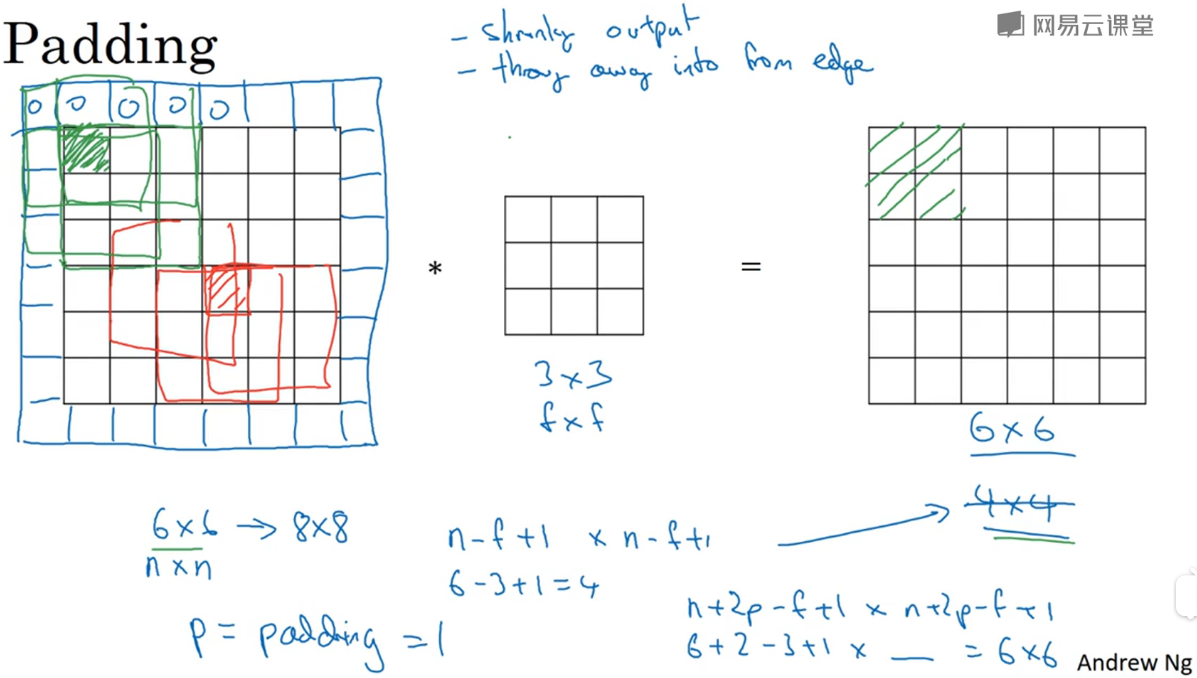n' = 8
( n' - f ) / s + 1 = ( 8 - 3 ) / 1 + 1 = 6

( n + 2p - f ) / s + 1

( n + 2p - f ) / s + 1 = n

p = (f − 1)/2

### 三维卷积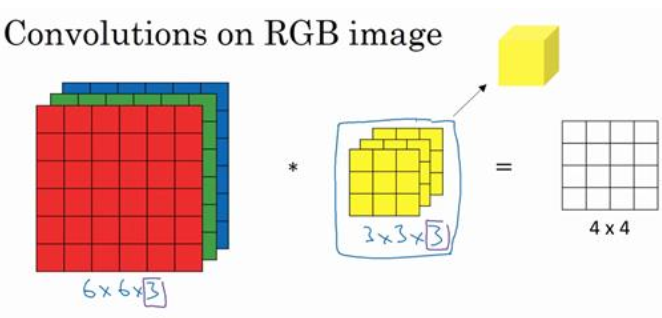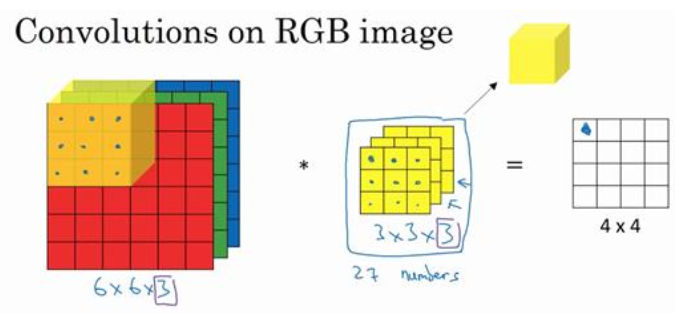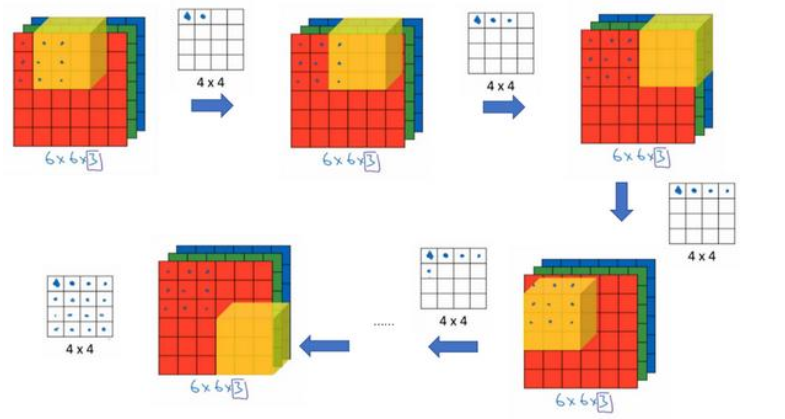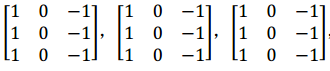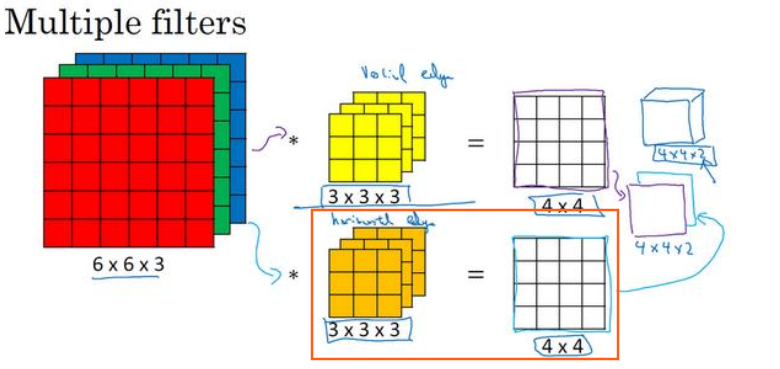nc 是 原图的通道数(3)，过滤器的通道数和原图的通道数必须一样，都是 nc。

nc' 是最终得到的图像的通道数，由使用的过滤器个数决定(而 nc' 又是下一层的输入图像的通道数)

### 卷积层全貌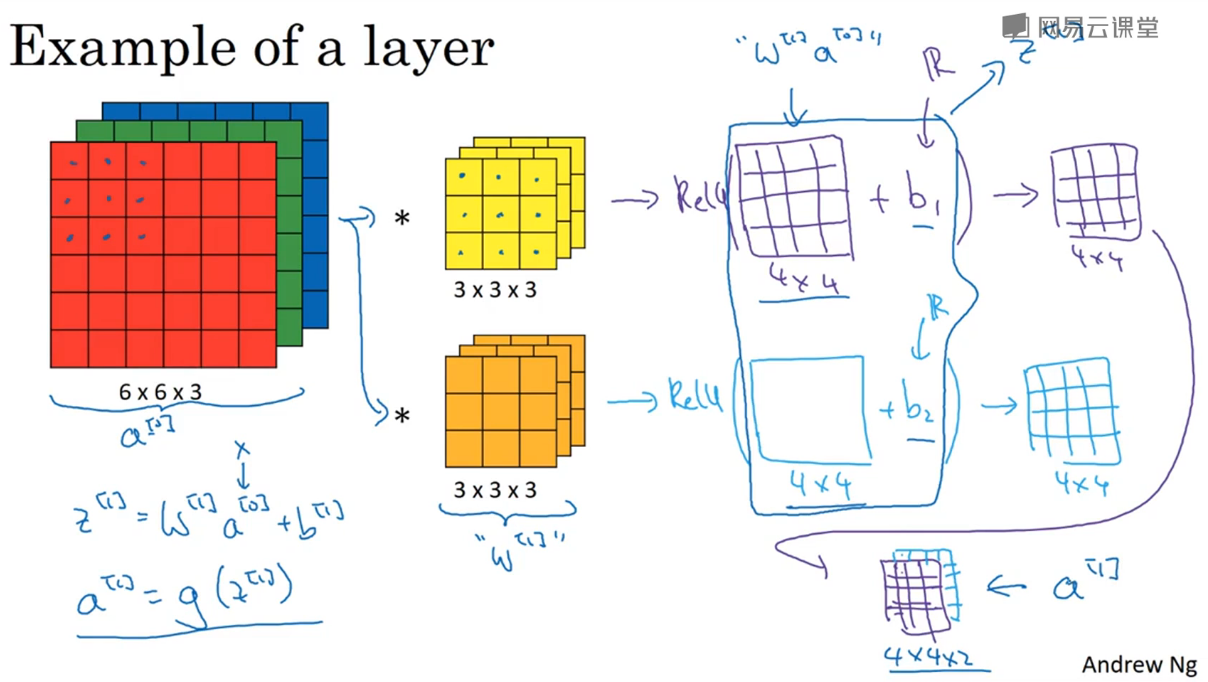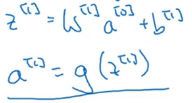• a^ 是我们原图的数据 X ，也就是上图 6x6x3 的RGB图。
• w^ 是这一层的权重矩阵，由 2 个 3x3x3 的过滤器组成。
• b^ 是第一层的偏置项，不同的过滤器对应不同的偏置值
• g() 是激活函数，本例子中使用 ReLu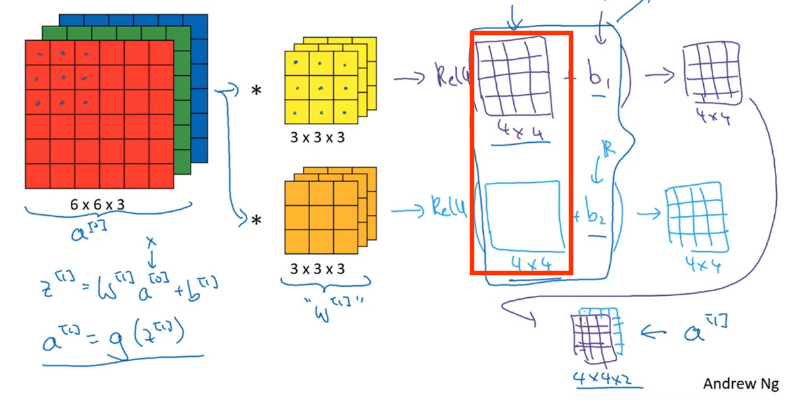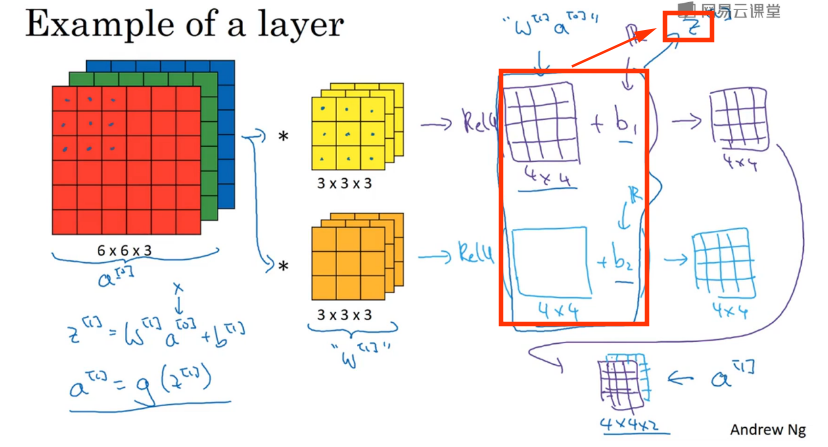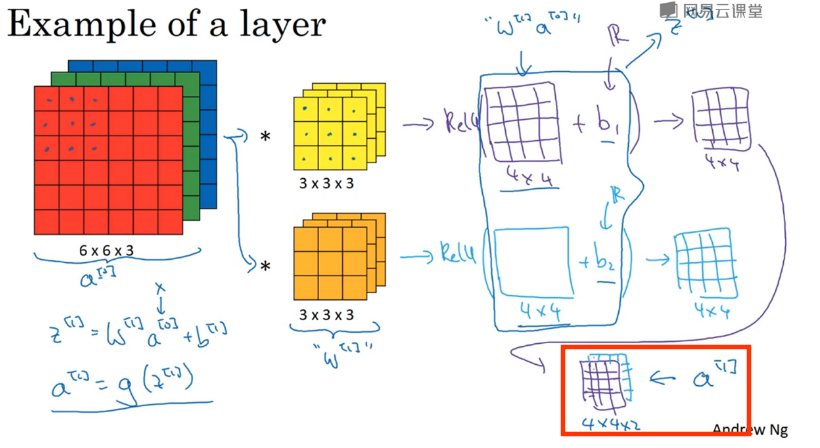### 为什么使用卷积

1. 稀疏连接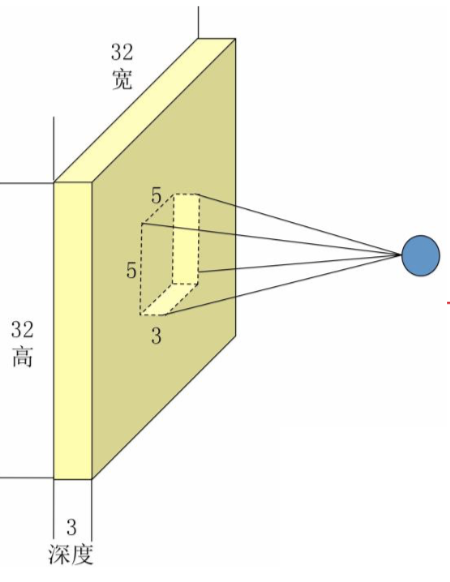2. 权值共享（参数共享）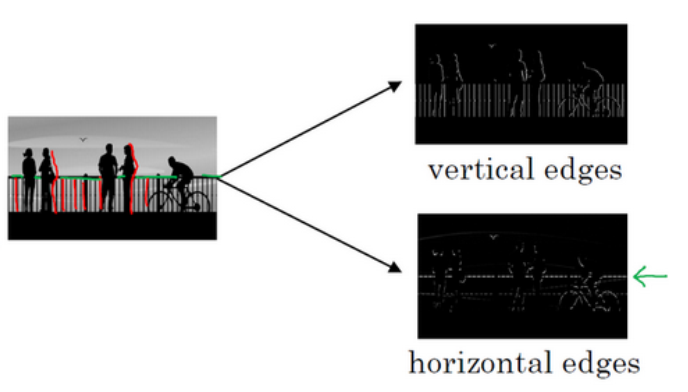【深度学习之美】局部连接来减参，权值共享肩并肩（入门系列之十一）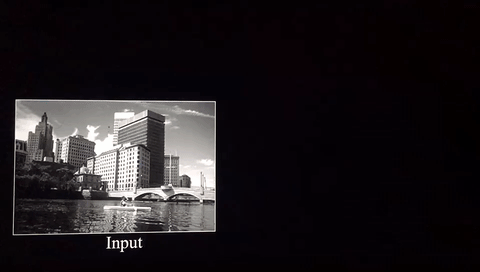## 采样层(Pooling Layer)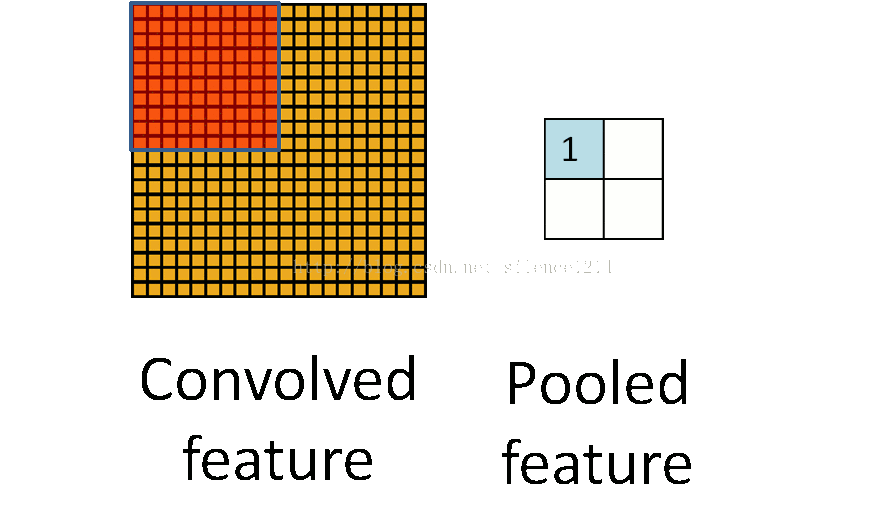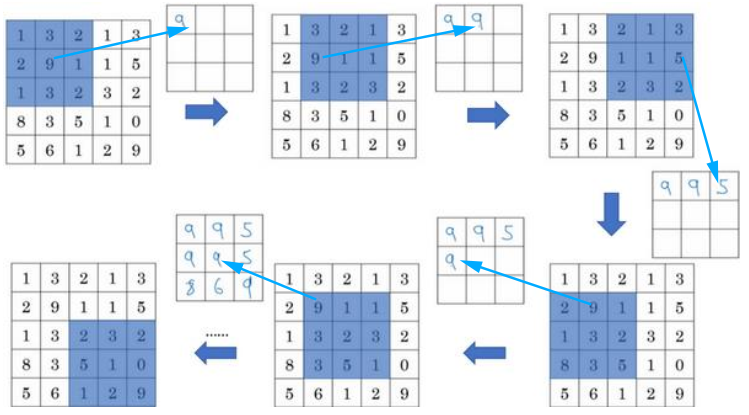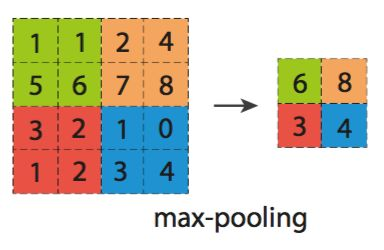## 全连接层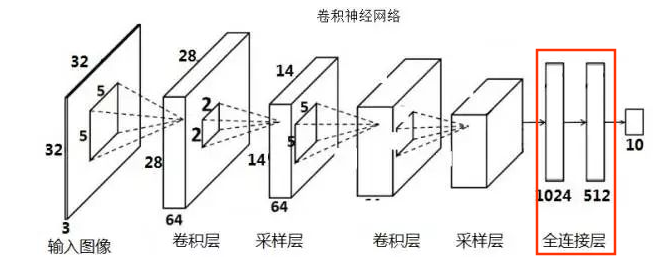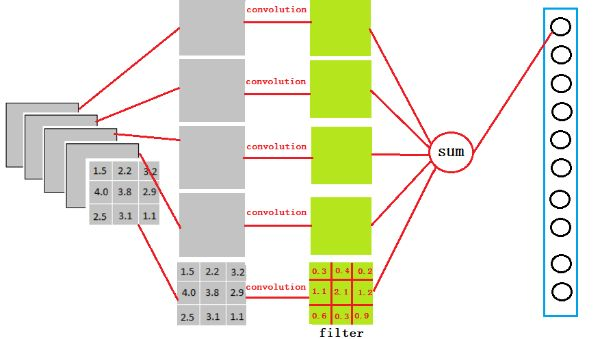1. 卷积层、采样层负责提取特征
2. 全连接层用于对提取到的特性进行分类